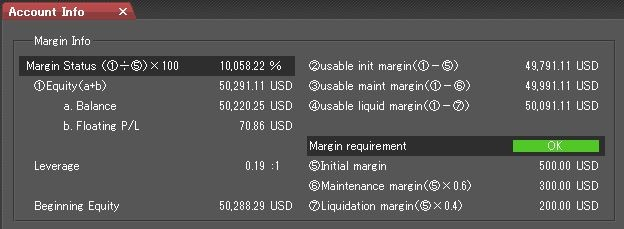# Checking account margin information

 No. Terms Definitions 1 Margin Status (Equity÷Initial Margin) X 100= [～%] If the account’s Margin Status is below 100%, you will not be able to execute new entry order. If the Margin Status is below 60%, margin call will occur. A fall to 40% will trigger liquidation of all open positions. 2 Equity The Total Sum of the Balance + Floating P/L + Cash Posting + Unrealized P/L + Unrealized Rollover. 3 Balance Total balance of the account. 4 Floating P/L Total P/L of open positions calculated on a mark-to-market basis. Account balance will only be affected when positions are liquidated. Account equity will continuously vary according to market valuation of the open positions. 5 Leverage (All Open Positions x Market Price)÷Equity= [～%] 6 Usable init margin (Usable initial margin) Equity – Initial Margin – Initial Margin on Order Displays unused margin for creating new entry order 7 Usable maint margin (Usable maintenance margin) Equity – Maintenance Margin 8 Usable liquid margin (Usable liquidation margin) Equity –Liquidation Margin. Displays margin for liquidation 9 Margin requirement The account’s margin status: [Normal]: Margin Status above 100% or no open positions. [Initial Margin Warning]: Margin Status between 60% and 100%. [Margin Call]: Margin Status between 40% and 60%. [Negative Balance]: Negative equity status. 10 Initial Margin Initial Margin = (All Open Positions x market price) x 5% Amount for 100% of initial margin requirement If the equity is below this amount, the status of margin requirement will turn to ‘Initial Margin Warning’ status. 11 Maint. Mr (Maintenance Margin) Amount for 60% of initial margin requirement. If the equity is below this amount, the status of margin requirement will turn to ‘Margin Call’ status. 12 Liquidation Mr (Liquidation Margin) Amount for 40% of initial margin requirement. If the equity is below this amount, all open positions will be liquidated. *Please note that calculation of account equity is performed in intervals and discrepancies from actual liquidation level may occur. 13 Beginning Equity Equity level of the previous day at New York Close.